﻿ 一种基于MATLAB的改进的水准网平差程序设计与实现 Design and Implementation of a Leveling Net Adjustment Program Based on MATLAB

Geomatics Science and Technology
Vol. 07  No. 04 ( 2019 ), Article ID: 32284 , 7 pages
10.12677/GST.2019.74024

Design and Implementation of a Leveling Net Adjustment Program Based on MATLAB

Liangliang Li, Henglin Guo, Lihua Wang

College of Geomatics, Shandong University of Science and Technology, Qingdao Shandong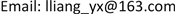Received: Aug. 31st, 2019; accepted: Sep. 16th, 2019; published: Sep. 23rd, 2019ABSTRACT

In order to calculate the leveling net data efficiently and accurately, by analyzing the data structure of leveling net, a further improvement is made to the adjustment program of MATLAB leveling net. Based on the principle of indirect adjustment, GUI function is used to implement leveling network adjustment. It solves the problem that in the general MATLAB adjustment program, it needs to manually replace the name to adjust. The application of real data in the experiment proves the correctness and practicability of the program, which has certain reference and guiding significance for MATLAB mapping data processing.

Keywords:Leveling Net Adjustment, Least Square Method, MATLAB1. 引言

MATLAB是一个强大的数学软件，其出色的矩阵处理能力，丰富的图形图像处理功能，以及简介化的程序语言为当今测绘领域提供了独具特色的研究手段。图形用户界面(Graphical User Interface, GUI)，是MATLAB的人机交互式界面设计功能。利用GUI设计可视化界面，解决测绘中的数据处理与平差问题是近几年的研究热点    。

2. 水准网间接平差原理

${V}^{\text{T}}PV=\mathrm{min}$ (1)

 (2)

$V=B\stackrel{^}{x}-l$ (3)

${N}_{BB}\stackrel{^}{x}-W=0$ (4)

$\stackrel{^}{x}={N}_{BB}^{-1}W$ (5)

3. 程序模块设计与实现

3.1. 可视化界面设计Figure 1. Interactive interface design

3.2. 数据的组织与录入

shift=num(:,1:2);

3.3. 概略高程计算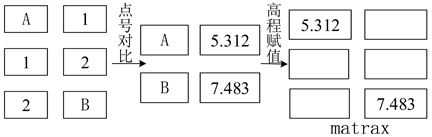Figure 2. Assignment method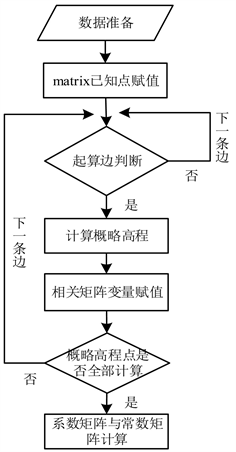Figure 3. General technical route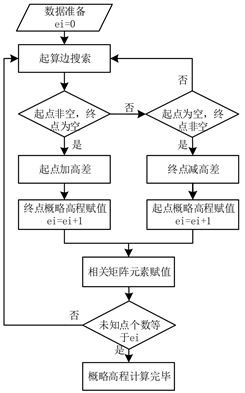Figure 4. Approximate elevation of unknown points

3.4. 法方程系数矩阵与常数阵的构建

4. 实验测试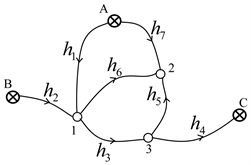Figure 5. Leveling network

5. 结束语

Design and Implementation of a Leveling Net Adjustment Program Based on MATLAB[J]. 测绘科学技术, 2019, 07(04): 179-185. https://doi.org/10.12677/GST.2019.74024

1. 1. 朱文武, 秦昆, 刘金钊, 等. 地震精密水准网平差的种高效算法研究[J]. 大地测量与地球动力学, 2018, 38(7): 695-697.

2. 2. 胡品. 测绘数据处理中MATLAB的优越性及应用[J]. 北京测绘, 2017(2): 150-153.

3. 3. 韩晓冬, 王浩森. 利用Matlab进行测绘数据处理分析[J]. 北京测绘, 2016(5): 133-136+127.

4. 4. 国家质量监督检验检疫总局. GB/T12898-2009国家三、四等水准测量规范[S]. 北京: 中国标准出版社, 2009.

5. 5. 李建章. 基于MATLAB的水准网平差程序设计[J]. 兰州交通大学学报, 2009, 28(3): 29-31.

6. 6. 王鹏磊, 刘长星. 基于MATLAB的水准网平差程序设计[J]. 测绘与空间地理信息, 2014, 37(3): 196-198.

7. 7. 武汉大学测绘学院测量平差学科组. 误差理论与测量平差基础[M]. 武汉: 武汉大学出版社, 2003.

8. 8. 胡奇. 水准网测量平差软件设计与开发[D]: [硕士学位论文]. 淮南: 安徽理工大学, 2012.

9. 9. 王建民, 谢锋珠. MATLAB与测绘数据处理[M]. 武汉: 武汉大学出版社, 2005.